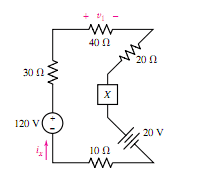# Power Being Absorbed

## Homework Statement

Find the power being absorbed by element X in Figure if it is a (a) 100 Ω resistor; (b) 40 V independent voltage source, + reference on top; (c) dependent voltage source labeled 25ix ,+ reference on top; (d) dependent voltage source labeled 0.8v1,+ reference on top; (e) 2 A independent current source, arrow directed upward.## Homework Equations

$$P = vi$$

## The Attempt at a Solution

a)

KVL:

$$20v + R_{1}i - 120v + R_{2}i + R_{3}i + R_{4}i + R_{5}i$$

$$i = \frac{20v - 120v}{R_{1} + R_{2} + R_{3} + R_{4} + R_{5}}$$

$$i = \frac{-100v}{200\Omega} = -0.5A$$

$$v = Ri = (100\Omega)(-0.5A) = -50v$$

$$P = (-50v)(-0.5A) = 25w$$

b)

KVL:

$$20v + R_{1}i - 120v + R_{2}i + R_{3}i + R_{4}i + 40v$$

$$i = \frac{20v + 40v - 120v}{R_{1} + R_{2} + R_{3} + R_{4}}$$

$$i = \frac{-60v}{100\Omega} = -0.6A$$

$$P = (40v)(-0.6A) = -24w$$

c)

KVL:

$$20v + R_{1}i - 120v + R_{2}i + R_{3}i + R_{4}i$$

$$i = \frac{20v - 120v}{R_{1} + R_{2} + R_{3} + R_{4}}$$

$$i = \frac{-100v}{100\Omega} = -1A$$

$$P = ((25)(-1A))(-1A) = 25w$$

d)

current is same as c)

$$i = -1A$$

$$v_{1} = (40\Omega)(-1A) = -40v$$

$$P = ((0.8)(-40v))(-1A) = 32w$$

e)

I am not sure how to do e) because I don't have a voltage and the current is 2A.

## Answers and Replies

Delphi51
Homework Helper
I did (a) and agree.
My take on (e) is that it is a current regulated supply that puts out whatever voltage it needs to push 2 A into the circuit. Assuming that the 120 V source has no internal resistance to having 2 A pushed backwards into it, you could calculate the 2 A source's potential V:
V = IR
20 + V - 120 = 2*100